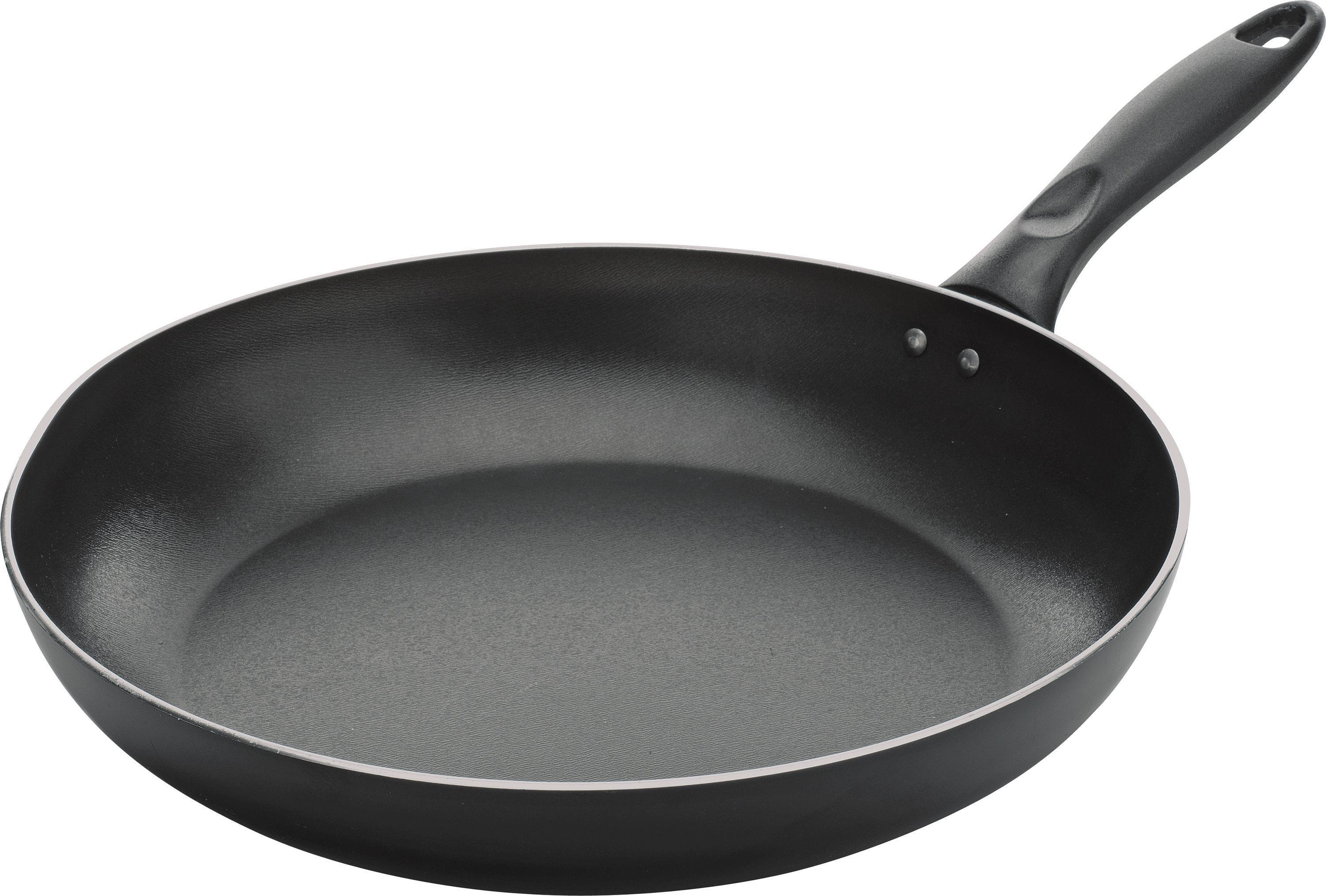# Advanced Practical Python #3: File Data Visualization

Ahoy Python Workshop’eneers! Time to head to uncharted waters with our data and attempt to graph it!

We will start by installing Seaborn through our command line/terminal:

`pip install seaborn`
`pip install git+https://github.com/mwaskom/seaborn.git`

Doing so will install all the requirments and Seaborn, notably “pandas” and “numpy.” Python’s Pandas is actually not pronounced like the cuddly herbivorous bear:But instead:+And it stands for “panel data” and has a wide range of other uses. We will also install “matplotlib” which is the math plotting library for Python:

`pip install matplotlib`

We could forgo Seaborn and use those three packages on their own (matplotlib, pandas, and numpy) but Seaborn provides us with prettier graphs and a more streamlined way to interact with our data.

With the installations out of the way, we will now start scripting!

Let’s start by bringing in Pandas for handling the data. For these imports we will be using an alias, so that we can type “pd” instead of typing in “pandas” each time we want to use pandas.

```# use pandas for data frame
import pandas as pd```

Now we’ll bring in matplotlib to customize our graphs:

```from matplotlib import pyplot as plt
```

Our last import will be the Seaborn module:

`import seaborn as sns`

Now let’s bring in our data set:

`df = pd.read_csv('filelist.csv')`

Here we named a variable called dataframe and used read_csv to open the “filelist.csv” csv file from our previous session.

Let’s look at our data using the head() command:

```result = df.head()
print "{}".format()```

What this does is pull the first 5 records of our dataset to see how it looks.

Using Pandas we can also do neat stuff like look at descriptive statistics of the data:

```result = df.describe()
print "{}".format(result)```

## Part 2 Time to Chart Our Data!

Now that we have a good grasp of how our data looks, we are going to create a neat little chart representing it.

We will first sort the data to go from largest to smallest by setting ascending to “False” on the field called “Number”:

`result = df.sort_values(by='Number',ascending=False)`

Now we will finally use Seaborn to graph the data:

`sns.barplot(x='File Types', y='Number', data=result)`

The syntax is pretty straightforward, where sns is Seaborn, barplot and chart type.

x = is the X-Axis, y= is the Y-Axis, and data=result selects the data.

We are able to alter the charts by adding various methods to it.

Since you might have a lot of file types, we can choose the top 10 results by adding an index location of 10:

`sns.barplot(x='File Types', y='Number', data=result).iloc[:10]`

If you run your code now… nothing will happen (unless you are using a Jupyter notebook)

What we need to add is:

`plt.show()`

And there you arrrrrre! Your chart should appear:

##If you got lost along the way, here is how your code should look:

```import pandas as pd
from matplotlib import pyplot as plt
import seaborn as sns

df = pd.read_csv('filelist.csv')

result = df.sort_values(by='Number',ascending=False)

sns.barplot(x='File Types', y='Number', data=result.iloc[:10],palette=colors)

plt.show()```

## Part 3. Customizing your graphs

You should add a title to your graph by adding the following line before plt.show():

`plt.title("File Frequencies")`

You can style your graphs too using Seaborn’s themes:

`sns.set_style('whitegrid')`

There are a total of 5 to choose from: `darkgrid`, `whitegrid`, `dark`, `white`, and `ticks`. But wait you want more?

You can also set your own colors, and then use the palette option:

```colors = ['#78C850',
'#F8D030',
'#E0C068']

sns.barplot(x='File Types', y='Number', data=result.iloc[:10],palette=colors)```

Finally, you can customize the x-axis and y-axis labels using .set(xlabel=’some x axis’, ylabel=’some y axis’) :

```sns.barplot(x='File Types', y='Number', data=result.iloc[:10],palette=colors).set(
xlabel='File Extension', ylabel='Frequency')
```

There’s a lot more you can do with Seaborn in addition to creating regression lines and histograms.

You can learn more about the types of Seaborn charts available by looking at the gallery below:Just a warning that the documentation on their website can be difficult to navigate!

Here is the final code:

```import pandas as pd
from matplotlib import pyplot as plt
import seaborn as sns

df = pd.read_csv('filelist.csv')

sns.set_style('whitegrid')

result = df.sort_values(by='Number',ascending=False)
print "{}".format(result)

colors = ['#78C850',
'#F8D030',
'#E0C068']

sns.barplot(x='File Types', y='Number', data=result.iloc[:10],palette=colors).set(
xlabel='File Extension', ylabel='Frequency')

# make sure the y limits start at 0
plt.ylim(0, None)
plt.title("File Frequencies")

plt.show()```

Onto the final tutorial: semantic analysis with Python!

## Extra Part

Time permitting, sail on over to the Los Angeles Open Data Portal and try to make some charts with the Los Angeles Police Department Crime Data:

https://data.lacity.org/A-Safe-City/Crime-Data-from-2010-to-Present/y8tr-7khq